## Odd Number

An Integer of the form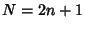, whereis an Integer. The odd numbers are therefore ...,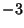,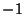, 1, 3, 5, 7, ... (Sloane's A005408), which are also the Gnomic Numbers. The Generating Function for the odd numbers is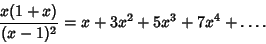Since the odd numbers leave a remainder of 1 when divided by two,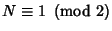for odd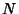. Integers which are not odd are called Even.

See also Even Number, Gnomic Number, Nicomachus's Theorem, Odd Number Theorem, Odd Prime

References

Sloane, N. J. A. Sequence A005408/M2400 in An On-Line Version of the Encyclopedia of Integer Sequences.'' http://www.research.att.com/~njas/sequences/eisonline.html and Sloane, N. J. A. and Plouffe, S. The Encyclopedia of Integer Sequences. San Diego: Academic Press, 1995.